##By Khan Academy

Sal shows how to group like terms to subtract and simplify radical terms, by simplifying the expression 4ï¿½ï¿½ï¿½ï¿½ï¿½ï¿½ï¿½ï¿½ï¿½ï¿½ï¿½ï¿½ï¿½ï¿½ï¿½ï¿½ï¿½ï¿½(81x^5)-2ï¿½ï¿½ï¿½ï¿½ï¿½ï¿½ï¿½ï¿½ï¿½ï¿½ï¿½ï¿½ï¿½ï¿½ï¿½ï¿½ï¿½ï¿½(81x^5)-ï¿½ï¿½ï¿½ï¿½ï¿½ï¿½ï¿½ï¿½ï¿½ï¿½ï¿½ï¿½ï¿½ï¿½ï¿½ï¿½ï¿½ï¿½(x^3).##By Khan Academy

Sal shows how to group like terms to add and simplify radical terms, by simplifying the expression ï¿½ï¿½ï¿½ï¿½ï¿½ï¿½ï¿½ï¿½ï¿½ï¿½ï¿½ï¿½ï¿½ï¿½ï¿½ï¿½ï¿½ï¿½(2x^2)+4ï¿½ï¿½ï¿½ï¿½ï¿½ï¿½ï¿½ï¿½ï¿½ï¿½ï¿½ï¿½ï¿½ï¿½ï¿½ï¿½ï¿½ï¿½8+3ï¿½ï¿½ï¿½ï¿½ï¿½ï¿½ï¿½ï¿½ï¿½ï¿½ï¿½ï¿½ï¿½ï¿½ï¿½ï¿½ï¿½ï¿½(2x^2)+ï¿½ï¿½ï¿½ï¿½ï¿½ï¿½ï¿½ï¿½ï¿½ï¿½ï¿½ï¿½ï¿½ï¿½ï¿½ï¿½ï¿½ï¿½8.# Math Antics - Multi-Digit Subtraction

##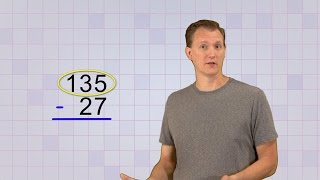By mathantics# Math Antics - Multi-Digit Multiplication Pt 1

##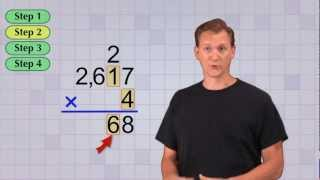By mathantics# Math Antics - Subtracting Mixed Numbers

##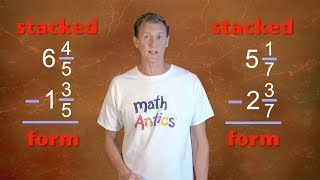By mathantics##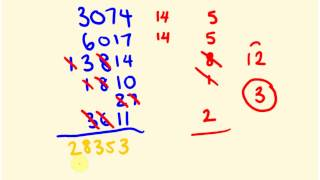By tecmath##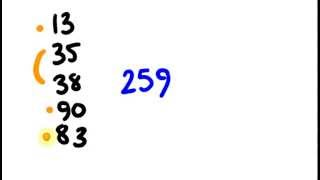By tecmath# Learn to Multiply Decimals (Decimal Multiplication) - 

##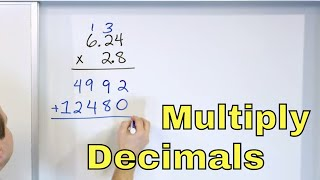By Math and Science# Even and Odd Numbers - Basic Introduction

##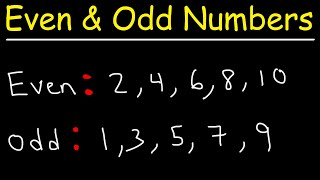By The Organic Chemistry Tutor# The Distributive Property In Arithmetic

##By mathantics# 08 - Learn Synthetic Division of Polynomials - Part 1

##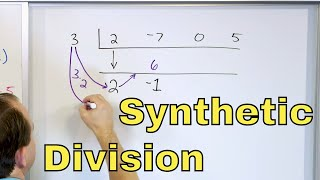By Math and Science

Quality Math And Science Videos that feature step-by-step example problems!# Math Antics - What Is Arithmetic?

##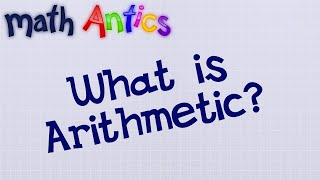By mathantics##By Anywhere Math# Math Antics - Decimal Arithmetic

##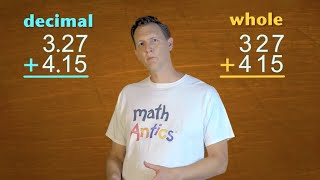By# Fast Math Trick to subtacting numbers mentally - two digit numbers

##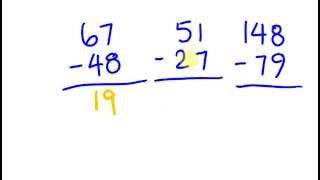By tecmath# 11 - Subtracting with Regrouping (Borrowing) - Part 1 (3rd Grade Math & 4th Grade Math)

##By Math and Science

Quality Math And Science Videos that feature step-by-step example problems!# Exciting Math for Kids

##By Homeschool Pop##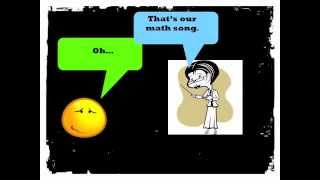By McCarthy Math Academy

With a little help from the group, Mindless Behavior, I have created a math video with lyrics to help my students to understand and apply core math skills for third grade. People of all ages can jam out to this one. Enjoy!

I've got a case of the operation blues.
Because I don't know which one I should use.
Look at the word problem for the clues.
The key words tell you how to choose.

Each means you multiply or you must divide.
Tryna find the total? Then you multiply
Total's in the problem? Then you must divide.
Not quick to solve it, draw it, get it right.

Addition's easy for me and you
Sum, In all, together, and total too.
When do you subtract? How many more?
Fewer? Left? Less? Difference in a score?

Place value's next. Disco on the " dess "
Ones, tens, hundreds, to the left
Thousands, Ten thousands, hundred...thousand
Say the name of the place, yeah.

The value's the amount of the place
For example, 2,060.
The value of the 2 is 2-0-0-0,
The value of the 6 is 6-0.

When you round, find and underline the place
Spotlight to the right, decide the digit's fate
5 or more, add 1 to the rounding place
4 or less, do nothing but walk away, (estimate)

A pen, penny is one, one cent
A Nic-kel is five, a dime is ten cents
25 for a quarter, George Washington
100 cents makes a dollar, there he goes again.

For pictographs, you gotta check out the key
One smiley face might really equal three
For bar graphs, pay attention to the scale
Think it's counting by ones, huh, you'll fail

Fractions are easy, just draw your best.
Here they go from least to greatest
1/12, 1/6, Â¼, 1/3,
Â½, 2/3, Â¾, Fraction nerd!

You see that number on top,
That's called the numerator
It describes the amount
That is being considered
And if you jump down from the fraction bar
Denominator
It's the total number of equal parts.

Let me give you an example:
Leslie Moin has some coins
A total of 9
2 happen to be pennies
While 7 are dimes.
What's the fraction of dimes?
How many coins? 9
How many dimes? 7
Say the fraction -- seven ninths

Length times width is Area
Distance around is Perimeter
Break down the GEOMETRY

3 sides makes triangle
5 pentagon, 6 hexagon
8 octagon, 10 decagon

Lines that never cross - PARALLEL
Lines that meet or cross - INTERSECTING
Lines that form right angles -- PERPENDICULAR

Same shape, same size -- CONGRUENT
Line that cuts in half - SYMMETRY
Up and Down - VERTICAL
Left to Right -- HORIZONTAL

An angle less than right - ACUTE
An angle opened wide - OBTUSE
Ninety degrees square corner - RIGHT ANGLE

Back to triangles
3 sides the same = equilateral
2 sides the same = isosceles
no sides the same = Hey, that's a scalene right!

So, that's it.
That's our math song.
Before we leave,
That way you know what the problem
Don't be lazy, be brilliant.
Piece! Like a fraction.# Learn to Divide Decimals (Long Division with Decimals) - 

##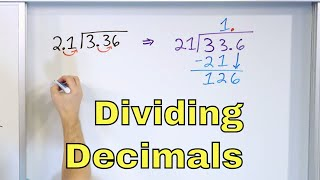By Math and Science# Math division - cast out nines trick

##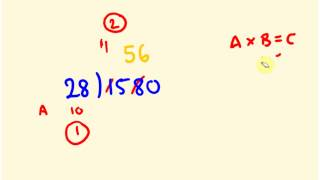By tecmath